Teaching mathematics

Start this free course now. Just create an account and sign in. Enrol and complete the course for a free statement of participation or digital badge if available.

Free course

# 2.1 Measuring scales

Reading a length from a ruler or tape measure is straightforward because these measuring devices are generally marked in unit lengths and each unit length is subdivided. A metre ruler may be marked in centimetres, each of which are subdivided into tenths or 0.1 of a centimetre.

The tape measure shown in Figure 2 has inches on one side, subdivided into quarters and sixteenths, and centimetres on the other. Note that the 10s figure for centimetres is only marked for every 10 centimetres.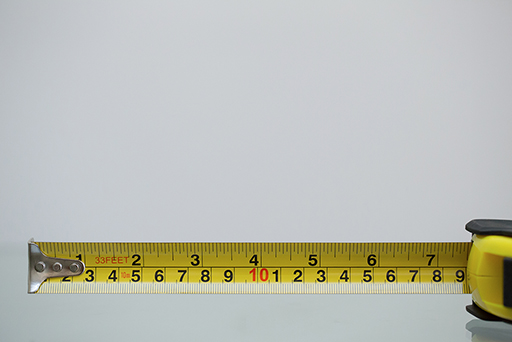Figure 2 A tape measure showing inches and centimetres

Reading temperature from thermometers is usually straightforward because they are marked in degrees Celsius (⁰C) and/or degrees Fahrenheit (⁰F) (Figure 3). However, although each gradation may be a unit length, they may be numbered in 20s, 10s or 5s.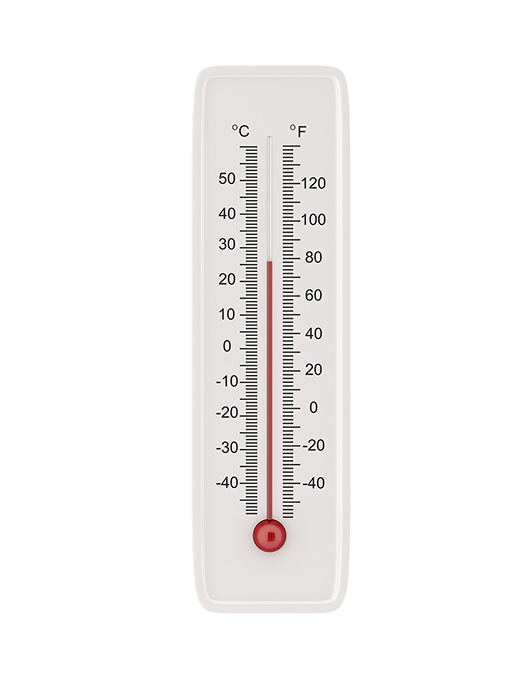Figure 3 A thermometer showing degrees Fahrenheit and Celsius

Reading a weight from a weighing scale may be more challenging. Some scales may be marked in kg or lb but the gradations might not be in the subunits. It may be necessary to count how many intervals a unit has been divided into and enact a division to find out what each subdivision represents. See the picture of the scales in Figure 4.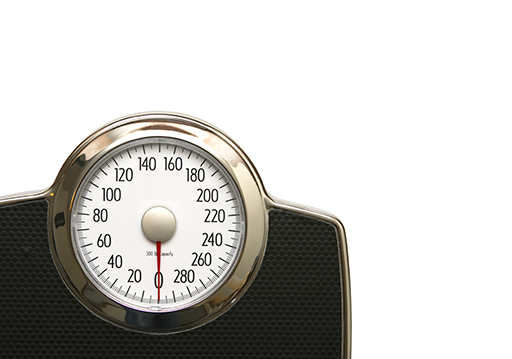Figure 4 An old-fashioned weighing machine

Reading the amount of fuel left in the tank of a car is a case in point. Fuel gauges rarely tell you how much fuel is in the tank. Instead, they tell you what fraction of a full tank is left (Figure 5).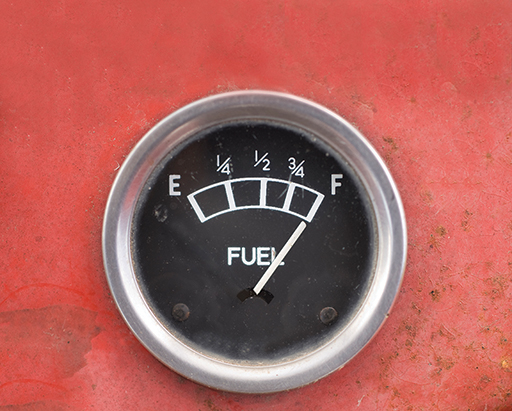Figure 5 A fuel gauge in a car

It is worth saying that many measuring tools are digital and provide a measurement in digits rather than on a scale. Time measurement provides a common example. We continue to measure time using analogue clocks, where the passage of time is reckoned by the position on the clock face of the hour-hand and the minute-hand (Figure 6). Yet we have become used to digital clocks which provide the time as a series of digits (Figure 7).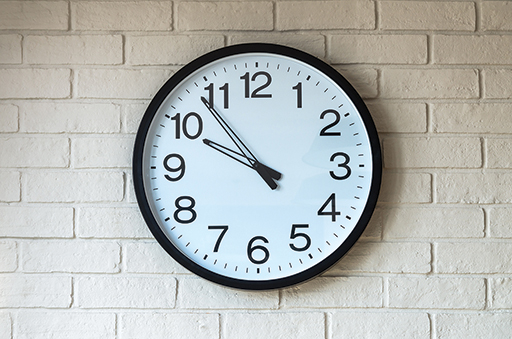Figure 6 An analogue clock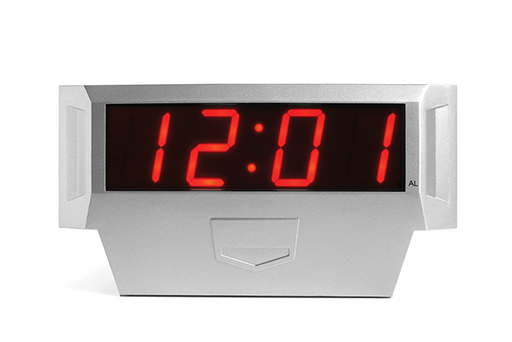Figure 7 A digital clock
TM_1